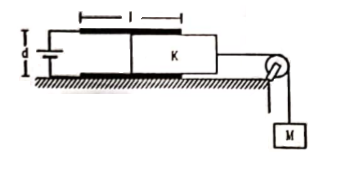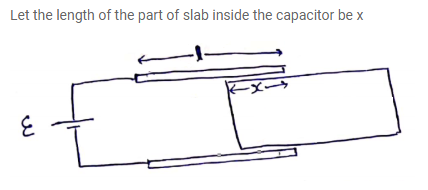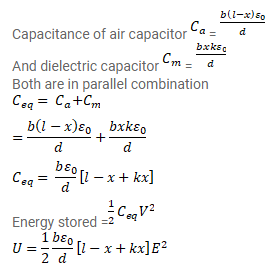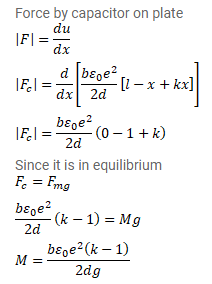# Consider the situation shown in figure.Question:

Consider the situation shown in figure. The width of each plate is $b$. The capacitor plates are rigidly clamped in the laboratory and connected to a battery of emf. All surface are frictionless. Calculate the value of $\mathrm{M}$ for which the dielectric slab will stay in equilibrium.Solution: